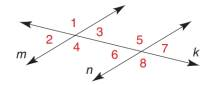Chapter 2.1, Problem 17E### Elementary Geometry for College St...

6th Edition
Daniel C. Alexander + 1 other
ISBN: 9781285195698

#### Solutions

Chapter
Section### Elementary Geometry for College St...

6th Edition
Daniel C. Alexander + 1 other
ISBN: 9781285195698
Textbook Problem
3 views

# Given: m ∥ n Transversal k m ∠ 3 = 6 x + y m ∠ 5 = 8 x + 2 y m ∠ 6 = 4 x + 7 y Find: x , y , and m ∠ 7Exercise 1 7 - 1 9

To determine

To find:

The values of x,y and the measure of the given angle.

Explanation

Definition:

The angles that are formed on opposite sides of the transversal and inside the two lines are alternate interior angles.

If the lines are parallel, then the alternate interior angles are equal.

If there exists two parallel lines cut by a transversal then the pair of interior angles on the same side of transversal is supplementary.

The pair of nonadjacent angles in the opposite direction that lie on two intersecting straight lines is called vertical angles.

Given:

The given figure is,

mn cut by a transversal k.

m3=6x+y, m5=8x+2y and m6=4x+7y.

The given angle is 7.

Calculation:

Consider the given figure,

From the figure, mn with transversal k.

3 and 6 are alternate interior angles.

m3=m66x+y=4x+7y6x4x=7yyx=3y.......(1)

From the figure, mn with transversal k.

3 and 5 are the interior angles on the same side of transversal k

### Still sussing out bartleby?

Check out a sample textbook solution.

See a sample solution

#### The Solution to Your Study Problems

Bartleby provides explanations to thousands of textbook problems written by our experts, many with advanced degrees!

Get Started

#### Rationalize the denominator: a. 2x3y b. xx4

Applied Calculus for the Managerial, Life, and Social Sciences: A Brief Approach

#### The slope of the tangent line to r = cos θ at is:

Study Guide for Stewart's Multivariable Calculus, 8th

#### Let and g(x) = x + 3. Then (g ∘ h)(x) = ______.

Study Guide for Stewart's Single Variable Calculus: Early Transcendentals, 8th